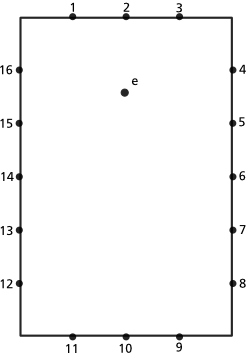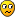Page 2 of 3

### Re: Synthesizing impulse responses

Posted: Thu Mar 20, 2008 1:13 am
Very simplified, sparse grid, assuming omni-directional emitter and receptor:16 wall nodes and one emitter.

1st order reflections
* For each wall node (1-16) calculate the distance between e and the node expressed in time (sample frames).
* For each wall node (1-16) calculate the level attenuated by the nodes absorption coefficient.
* For each wall node (1-16) calculate the angle of incidence.

1st order rendering
* For each wall node (1-16) calculate the distance between receptor and the node expressed in time (sample frames).
* For each wall node (1-16) compare the stored angle of incidence with the receptors angle of incidence. If equal then record the response with the nodes stored level at summed time.

2nd order reflections
Is calculated the same way as the 1st order rendering but with each node acting as a receptor and the stored 1st order values and nodes are used as emitters.

2nd order rendering
Is calculated just like the 1st order rendering but using the result of the 2nd order reflection step instead of the 1st.

Of course, if a receptor don't have a free line-of-sight to an emitter (or nodes acting as receptor/emitters), for example they belong to the same wall, that pair shouldn't be calculated.

All the reflection steps (1st, 2nd and so on) are calculated first then the final impulse is rendered using them as input, so that the result of the reflection steps can be reused to render multi-channel impulses. I just interleaved the steps to make the explanation clearer (I hope!).

Is this completely bonkers?

### Re: Synthesizing impulse responses

Posted: Thu Mar 20, 2008 6:55 pm
OK, maybe the radiosity-like approach described above is a bit overkill. I've been figuring out the trigonometry for calculating the first, second and third order reflections, not a math wiz really.### Re: Synthesizing impulse responses

Posted: Fri Mar 21, 2008 1:48 pm
I'm starting to get IRs by intuition. Here's a fun experiment anyone can do:

* Open up your favorite sound editor.
* Create an empty one second mono file.
* Use the draw tool to draw single samples.
* Draw one or two samples with big positive values far apart.
* Save it as WAV.
* Load it into a convolution reverb (do an equal dry/wet mix) and play something like a piano through it: the effect will most likely be of a delay echo.
* Go on and manually create new IRs and play with the convolution.

Of course the result is what you expect if you understand plotting IRs with pen and paper to analyze a room.

* If we only draw one sample at 0.5s the result will be a 0.5s delay of the sound in the output from the convolution.
* If we draw two samples, at 0.5s and 0.8s and the sound we play decay in less than 0.3s, the result will be a repetition (the sound delayed to 0.5s respective 0.8s) in the output from the convolution.
* If we draw a sample, at 0.05s and the sound we play is sustained at least 0.05s and we sum the output from the convolution with the direct sound, the result will be a self-filtration of the sound (sample at 0.0s and 0.05s in the sound will be summed together, sample at 0.01s and 0.06s likewise and so on).
** So If I want to filter a specific frequency I need to calculate the distance between the direct sound and the IR sample for that frequency. For a 1Hz tone (yes, I know, not audible but just an example) that would be 1s and a sample with positive value 1s into the IR will boost that frequency while a sample with a negative value will cut it?
** The limits would be 2 samples apart for 20 kHz and 2205 samples for 20 Hz at a sample rate of 44.1 kHz.

Edit: Clarified some of my reasoning.

So IRs are just comb filters on steroids?

### Re: Synthesizing impulse responses

Posted: Fri Mar 21, 2008 4:02 pm
That's not a bad description. You might also think of them as being custom comb filters specifically modeled on a room or a piece of hardware, since, in theory anyway, a bunch of comb filters can do anything any other kind of filter can do (assuming you make your comb filters with very short delays). It's all just clever manipulation of delay lines in the end. The difference is, a room's impulse response is ready-made for convolution, whereas the impulse response from a piece of gear like an EQ, a microphone, or a guitar cabinet, needs a bit of massaging first due to how it's generated.

I'm not that great an expert, but this is what I gleaned from reading my copy of the Csound Book, which is chock-full of DSP theory written in an accessible way.

### Re: Synthesizing impulse responses

Posted: Fri Mar 21, 2008 4:05 pm
Yes, I should give the Csound book a good read (only previously browsed it). But it's fascinating how easily you can synthesize various IRs and get great sounding result while not necessarily simulating anything particular.

### Re: Synthesizing impulse responses

Posted: Fri Mar 21, 2008 4:58 pm
You just gave me an interesting idea about how to model/simulate a guitar cabinet, actually. It involves a pair of very short comb filters plus the IR response of some birch plywood. I'll post more later, as I now need to drive down to another university to see about transferring in next year for my degree.

### Re: Synthesizing impulse responses

Posted: Fri Mar 21, 2008 5:16 pm
A fun application would be to let the user draw the impulse response in a waveform monitor, generate a input tone in another waveform monitor (like a sinus wave of a specific frequency) and show the convolved output in a third waveform monitor.

### Re: Synthesizing impulse responses

Posted: Fri Mar 21, 2008 6:36 pm
* Just to keep a reminder of it somewhere:

velocity = wavelength x frequency,
344 m/s = 2.75 m x 125 Hz

wavelength = velocity / frequency,
2.75 m = 344 m/s / 125 Hz

frequency = velocity / wavelength,
125 Hz = 344 m/s / 2.75 m

period = 1 / frequency,
0.008 s = 1 / 125 Hz

period = samplerate / frequency,
352 samples = 44100 Hz / 125 Hz

* The velocity of sound in air is 344 m/s at 21 °C.

* The velocity of sound in fresh water is 1482 m/s at 20 °C.

* The velocity of sound in vacuum is 0 m/s.

* 1 dB is smallest perceivable level change (for pure tones).

* An increase of 10 dB is perceived as a doubling of loudness.

* The audible range of sound is 20 Hz to 20 kHz.

* The Nyquist frequency is half the sampling frequency.

* Sound intensity from a point source of sound will obey the inverse square law.

* Two traveling waves which exist in the same medium will interfere with each other (constructive/destructive interference).

* Sound waves in air are longitudinal waves.

* Auditorium acoustics guide.

(That HyperPhysics site is gold. Kudos to Lowkey who made me google "refraction of sound" that led me to it!)### Re: Synthesizing impulse responses

Posted: Fri Mar 21, 2008 11:18 pm
I just commented on the speaker/cabinet simulation bit in my original amp sim thread, so I don't take this one off-topic, which I am now doing with this post.Oh, those are handy equations to have. Thanks!

(There, back on-topic.)### Re: Synthesizing impulse responses

Posted: Sat Mar 22, 2008 12:10 am
Could light refraction formale be used? Although light is faster than sound they are still waveforms.